# Maharashtra Board Class 9 Maths Solutions Chapter 5 Linear Equations in Two Variables Practice Set 5.2

## Maharashtra State Board Class 9 Maths Solutions Chapter 5 Linear Equations in Two Variables Practice Set 5.2

Question 1.
In an envelope there are some ₹5 notes and some ₹10 notes. Total amount of these notes together is ₹350. Number of ₹5 notes are less by 10 than twice the number of ₹10 notes. Then find the number of ₹5 and ₹10 notes.
Solution:
Let the number of ₹5 notes be ‘x’ and the number of ₹10 notes be ‘y’
Total amount of x notes of ₹ 5 = ₹ 5x
Total amount ofy notes of ₹ 10 = ₹ 10y
∴ Total amount = 5x + 10y
According to the first condition,
total amount of the notes together is ₹350.
∴ 5x + 10y = 350 …(i)
According to the second condition,
Number of ₹ 5 notes are less by 10 than twice the number of ₹ 10 notes.
∴ x = 2y – 10
∴ x – 2y = -10 …..(ii)
Multiplying equation (ii) by 5,
5x – 10y = -50 …(iii)
5x + 10y =350
+ 5x – 10y = -50
10x =300
∴ x = $$\frac { 300 }{ 10 }$$
∴ x = 30
Substituting x = 30 in equation (ii),
x – 2y = -10
30 – 2y = -10
∴ 30 + 10 = 2y
∴ 40 = 2y
∴ y = $$\frac { 40 }{ 2 }$$
∴ y = 20
There are 30 notes of ₹ 5 and 20 notes of ₹ 10 in the envelope.

Question 2.
The denominator of a fraction is 1 less than twice its numerator. If 1 is added to numerator and denominator respectively, the ratio of numerator to denominator is 3 : 5. Find the fraction.
Solution:
Let the numerator of the fraction be ‘x’ and its denominator be ‘y’.
Then, the required fraction is $$\frac { x }{ y }$$ .
According to the first condition,
the denominator is 1 less than twice its numerator.
∴ y = 2x – 1
∴ 2x – y = 1 …(i)
According to the second condition,
if 1 is added to the numerator and the denominator, the ratio of numerator to denominator is 3 : 5.
∴ $$\frac { x+1 }{ y+1 }$$ = $$\frac { 3 }{ 5 }$$
∴ y + 1 = 5
∴ 5(x + 1) = 3(y + 1)
∴ 5x + 5 = 3y + 3
∴ 5x – 3y = 3 – 5
∴ 5x – 3y = -2 ……(ii)
Multiplying equation (i) by 3,
6x – 3y = 3 …(iii)
Subtracting equation (ii) from (iii),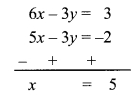Substituting x = 5 in equation (i),
∴ 2x – y = 1
∴ 2(5) – y = 1
∴ 10 – y = 1
∴ y= 10 – 1 =9
∴ The required fraction is $$\frac { 5 }{ 9 }$$.

Question 3.
The sum of ages of Priyanka and Deepika is 34 years. Priyanka is elder to Deepika by 6 years. Then find their present ages.
Solution:
Let the present age of Priyanka be ‘x’ years and that of Deepika be ‘y’ years.
According to the first condition,
Priyanka’s age + Deepika’s age = 34 years
∴ x + y = 34 …(i)
According to the second condition,
Priyanka is elder to Deepika by 6 years.
∴ x =y + 6
∴ x – y = 6 …..(ii)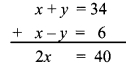∴ x = 20
Substituting x = 20 in equation (i),
x + y = 34
∴ 20 + y = 34
∴ y = 34 -20= 14
∴ The present age of Priyanka is 20 years and that of Deepika is 14 years.

Question 4.
The total number of lions and peacocks in a certain zoo is 50. The total number of their legs is 140. Then find the number of lions and peacocks in the zoo.
Solution:
Let the number of lions in the zoo be ‘x’ and the number of peacocks be ‘y’.
According to the first condition,
the total number of lions and peacocks is 50.
∴ x + y = 50 …(i)
Lion has 4 legs and Peacock has 2 legs.
According to the second condition,
the total number of their legs is 140.
∴ 4x + 2y = 140
Dividing both sides by 2,
2x + y = 70 …(ii)
Subtracting equation (i) from (ii),Substituting x = 20 in equation (i),
x + y = 50
∴ 20 + y = 50
∴ y = 50 – 20 = 30
∴ The number of lions and peacocks in the zoo are 20 and 30 respectively.

Question 5.
Sanjay gets fixed monthly income. Every year there is a certain increment in his salary. After 4 years, his monthly salary was ₹ 4500 and after 10 years his monthly salary became ₹ 5400, then find his original salary and yearly increment.
Solution:
Let the original salary of Sanjay be ₹ ‘x’ and his yearly increment be ₹ ‘y’.
According to the first condition, after 4 years his monthly salary was ₹ 4500
∴ x + 4y = 4500 …..(i)
According to the second condition,
after 10 years his monthly salary became ₹ 5400
∴ x + 10y = 5400 …(ii)
Subtracting equation (i) from (ii),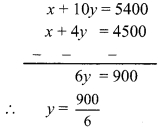∴ y = 150
Substituting y = 150 in equation (i),
x + 4y = 4500
∴ x +4(150) = 4500
∴ x + 600 = 4500
∴ x = 4500 – 600 = 3900
∴ The original salary of Sanjay is ₹ 3900 and his yearly increment is ₹ 150.

Question 6.
The price of 3 chairs and 2 tables is ₹ 4500 and price of 5 chairs and 3 tables is ₹ 7000, then find the price of 2 chairs and 2 tables.
Solution:
Let the price of one chair be ₹ ‘x’ and that of one table be ₹ ‘y’.
According to the first condition,
the price of 3 chairs and 2 tables is ₹ 4500
∴ 3x + 2y = 4500 ,..(i)
According to the second condition, the price of 5 chairs and 3 tables is ? 7000
∴ 5x + 3y = 7000 …(ii)
Multiplying equation (i) by 3,
9x + 6y = 13500 ….(iii)
Multiplying equation (ii) by 2,
10x + 6y= 14000 …(iv)
Subtracting equation (iii) from (iv),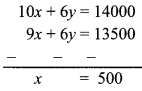Substituting x = 500 in equation (i),
3x + 2y = 4500
∴ 3(500)+ 2y = 4500
∴ 1500 + 2y = 4500
∴ 2y = 4500- 1500
∴ 2y = 3000
∴ y = $$\frac { 3000 }{ 2 }$$
∴ y = 1500
∴ Price of 2 chairs and 2 tables = 2x + 2y
= 2(500)+ 2(1500)
= 1000 + 3000 = ₹ 4000
∴ The price of 2 chairs and 2 tables is ₹ 4000.

Question 7.
The sum of the digits in a two-digit number is 9. The number obtained by interchanging the digits exceeds the original number by 27. Find the two-digit number.
Solution:
Let the digit in unit’s place be ‘x’ and the digit in ten’s place be ‘y’.According to the first condition.
the sum of the digits in a two-digit number is 9
x + y = 9 …(i)
According to the second condition,
the number obtained by interchanging the digits exceeds the original number by 27
∴ 10x + y = 10y + x + 27
∴ 10x – x + y – 10y = 27
∴ 9x – 9y = 27
Dividing both sides by 9,
x – y = 3 …….(ii)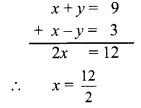∴ x = 6
Substituting x = 6 in equation (i),
x + y = 9
∴ 6 + y = 9
∴ y = 9 – 6 = 3
∴ Original number = 10y + x = 10(3)+ 6
= 30 + 6 = 36
∴ The two digit number is 36.

Question 8.
In ∆ABC, the measure of ∠A is equal to the sum of the measures of ∠B and ∠C. Also the ratio of measures of ∠B and ∠C is 4 : 5. Then find the measures of angles of the triangle.
Solution:
Let the measure of ∠B be ‘x°’ and that of ∠C be ‘y°’.
According to the first condition,
m∠A = m∠B + m∠C
∴ m∠A = x° + y°
In AABC,
m∠A + m∠B + m∠C = 180° …[Sum of the measures of the angles of a triangle is 180°]
∴ x + y + x + y = 180 ,
∴ 2x + 2y = 180
Dividing both sides by 2,
x + y = 90 …(i)
According to the second condition,
the ratio of the measures of ∠B and ∠C is 4 : 5.
∴ $$\frac { x }{ y }$$ = $$\frac { 4 }{ 5 }$$
∴ 5x = 4y
∴ 5x – 4y = 0 …….(ii)
Multiplying equation (i) by 4,
4x + 4y = 360 …(iii)∴ x = 40
Substituting x = 40 in equation (i),
x + y = 90
∴ 40 + y = 90
∴ y = 90 – 40
∴ y = 50
∴ m∠A = x° + y° = 40° + 50° = 90°
∴ The measures of ∠A, ∠B and ∠C are 90°, 40°, and 50° respectively.

Question 9.
Divide a rope of length 560 cm into 2 parts such that twice the length of the smaller part is equal to $$\frac { 1 }{ 3 }$$ of the larger part. Then find the length of the larger part.
Solution:
Let the length of the smaller part of the rope be ‘x’ cm and that of the larger part be ‘y’ cm.
According to the first condition,
total length of the rope is 560 cm.
∴ x + y = 560 …(i)
Twice the length of the smaller part = 2x
$$\frac { 1 }{ 3 }$$rd length of the larger part = $$\frac { 1 }{ 3 }$$y
According to the second condition,
2x = $$\frac { 1 }{ 3 }$$ 3
∴ 6x = y
∴ 6x – y = 0 ……(ii)∴ x = 80
Substituting x = 80 in equation (ii),
6x – y = 0
∴ 6(80) – y = 0
∴ 480 – y = 0
∴ y = 480
∴ The length of the larger part of the rope is 480 cm.

Question 10.
In a competitive examination, there were 60 questions. The correct answer would carry 2 marks, and for incorrect answer 1 mark would be subtracted. Yashwant had attempted all the questions and he got total 90 marks. Then how many questions he got wrong?
Solution:
Let us suppose that Yashwant got ‘x’ questions right and ‘y’ questions wrong.
According to the first condition, total number of questions in the examination are 60.
∴ x + y = 60 …(i)
Yashwant got 2 marks for each correct answer and 1 mark was deducted for each wrong answer.
∴ He got 2x – y marks.
According to the second condition,
he got 90 marks.
2x – y = 90 … (ii)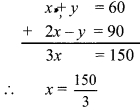∴ x = 50
Substituting x = 50 in equation (i),
50 + y = 60
∴ y = 60 – 50 = 10
∴ Yashwant got 10 questions wrong.

Maharashtra Board Class 9 Maths Chapter 5 Linear Equations in Two Variables Practice Set 5.2 Intext Questions and Activities

Question 1.
The population of a certain town was 50,000. In a year, male population was increased by 5% and female population was increased by 3%. Now the population became 52020. Then what was the number of males and females in the previous year? (Textbook pg. no. 89)
Solution:
Step 1: Read the given word problem carefully and try to understand it.

Step 2: Make assumptions using two variables x and y.
Let the number of males in previous year be
‘x’ and the number of females be ‘y’.

Step 3: From the given information, form mathematical statements using the above variables.
According to the first condition,
the total population of town was 50,000.
∴ x + y = 50000 …(i)
Male population increased by 5%.
∴ Number of males = x + 5% of x , 5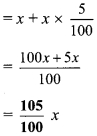Female population increased by 3%.
∴ Number of females = y + 3% of yAccording to the second condition,
in a year population became 52020
∴ $$\frac{105}{100} x+\frac{103}{100} y=52020$$
∴ 105 x + 103 y = 5202000 …(ii)
Multiplying equation (i) by 103,
103 x + 103 y = 5150000 …(iii)

Step 4: Here, we use elimination method.
Subtracting equation (iii) from (ii),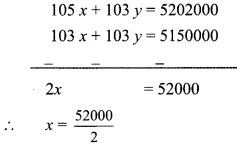∴ x = 26000
Substituting x = 26000 in equation (i),
∴ 26000 + y = 50000
∴ y = 50000 – 26000
∴ y = 24000
∴ Number of males = x = 26000
∴ Number of females = y = 24000